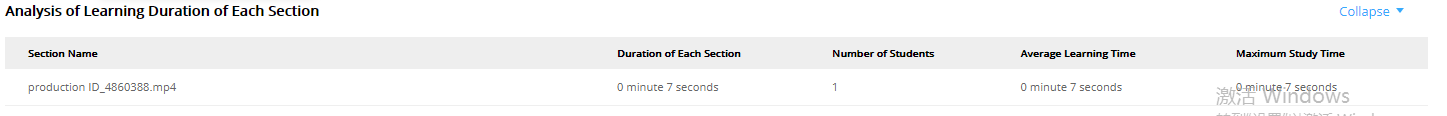# lms online courseware management

## How to view statistical analysis of lms training courses

Statistical analysis of LMS training courses can see detailed analysis data, including a summary of LMS training courses, analysis of the number of learners in chapters, statistical analysis of categories, and analysis of chapter learning time.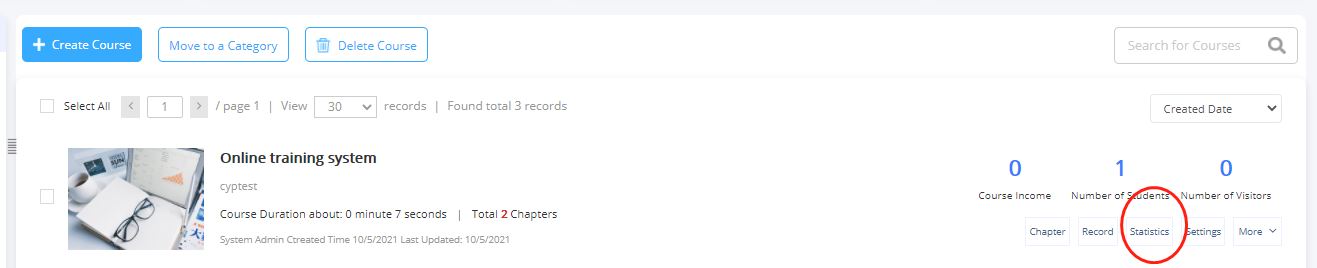1. Summary of LMS training courses: You can see the number of learners, average learning time, and percentage of learners of the course.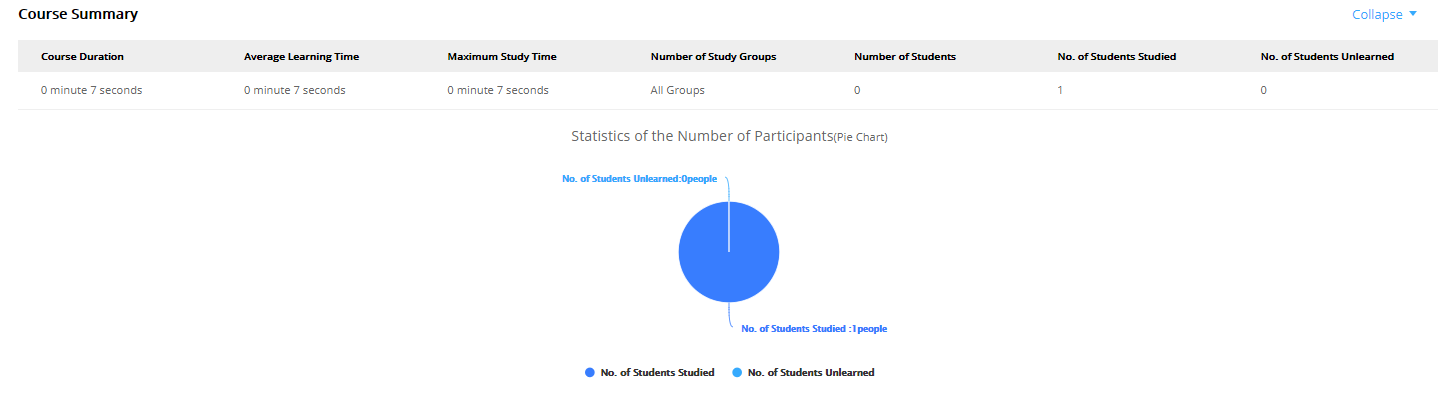2 Analysis of the number of learners in chapters: You can see the number of learners in each chapter.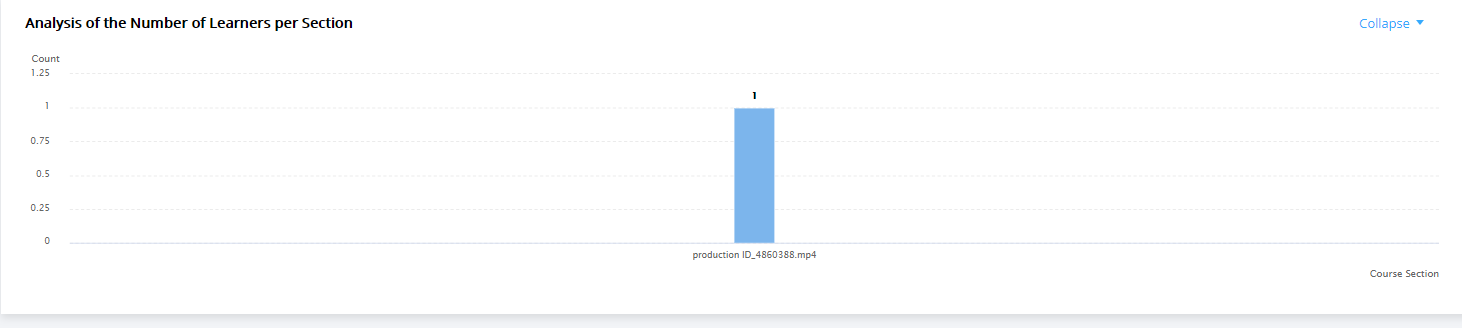3. Categorical Statistical Analysis: You can see the number of learners in each group, the number of completions, and the test scores.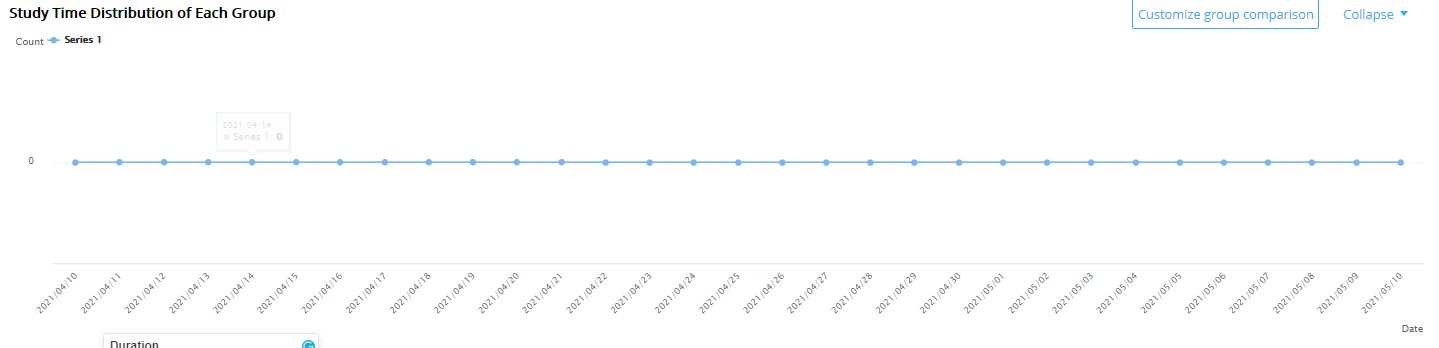4. Chapter study time analysis: You can see the study time, number of people, average study time, and maximum study time of each chapter.